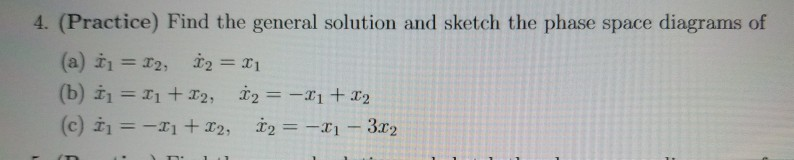# (Solved): Find The General Solution Just B And C Is Fine. Please Try To Include A Somewhat Detailed Step Solut...

Find the general solutionJust b and c is fine. Please try to include a somewhat detailed step solution. Asking as I do not understand the given solution. No diagram sketching needed. Thanks

4. (Practice) Find the general solution and sketch the phase space diagrams of (a) t1 = 12, 12 = ri (b) 11 = I1 + 12, 12 = -21 +22 (c) i = -11 + x2 12 = -x - 3x2 . :

We have an Answer from Expert